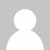In today’s Advent of Code puzzle, we found ourselves needing to interpret a program. This has been a recurring theme over the years, and so the steps to solve it were familiar. First, parse the instructions, then, run the instructions, and finally, extract the answer to each part of the puzzle.

### Parsing with RegEx

The instructions in our puzzle input took the following form:

``````sg inc 242 if kmh <= 9
``````

In this example, if register `khm` is less than or equal to 9, increment the value of register `sg` by 242. Here’s my rough and ready regex that I used to parse each instruction into a simple object:

``````function parseInstruction(instruction) {
let g = /(\w+) (\w+) (-?\d+) if (\w+) ([<>=!]+) (-?\d+)/.exec(instruction)
return { target: g, op: g, amount: Number(g), testReg: g, testOp: g, testAmount: Number(g) };
}
``````

### Running the Instructions

To run the instructions we needed to store the state of all the registers. I opted once again for the ES6 `Map`. I passed the empty map into my `executeInstructions` function which looped through each instruction executing each instruction. I made a helper to test if the condition was met, `testRegister`, and another helper, `applyOp`, to apply the operation to a register.

``````function executeInstructions(registers, instructions) {
for (let i of instructions) {
if (testRegister(registers.get(i.testReg) || 0, i.testOp, i.testAmount)) {
registers.set(i.target, applyOp(registers.get(i.target) || 0, i.op, i.amount));
}
}
}
``````

Once again, in JavaScript land, it’s easier to mutate our state than go for a fully functional approach. If we had an immutable map type available to us, then that could easily be used here.

To cope with registers that are not yet set in the map, we can use the shorthand `registers.get(i.testReg) || 0` syntax to return 0 if the key is not found in the map.

The initial helper functions `testRegister` and `applyOp` I created looked like this:

``````function testRegister(value, op, amount) {
switch(op) {
case '<': return value < amount;
case '>': return value > amount;
case '<=': return value <= amount;
case '>=': return value >= amount;
case '!=': return value !== amount;
case '==': return value === amount;
default: throw new Error("invalid test op " + op)
}
}

function applyOp(value, op, amount) {
switch(op) {
case 'inc': return value + amount;
case 'dec': return value - amount;
default: throw new Error("invalid op " + op)
}
}
``````

### Solving the Puzzle

The answer to part 1 was just to find the maximum register value. This can be done using the `max` helper function I created for an earlier puzzle to find the maximum value

``````let part1 = max(registers.values())
``````

For part 2, we were required to return the largest value that had been in any register. This is easily tracked in the `executeInstructions` function just before we set the register value

``````let newValue = applyOp(registers.get(i.target) || 0, i.op, i.amount)
largest = Math.max(largest,newValue)
registers.set(i.target, newValue);
``````

### Refactoring

Once I’ve solved an Advent of Code puzzle, if time permits I like to refactor my solution a bit. I’m not trying to “code golf” it, but often simplifications are possible.

For example, the `testRegister` and `applyOp` functions are very similar, and could be combined into one, using a lookup that works both for the conditional tests and increment or decrements:

``````const ops = {'<':(a,b)=>a<b,'>':(a,b)=>a>b,'<=':(a,b)=>a<=b,'>=':(a,b)=>a>=b,'!=':(a,b)=>a!==b,'==':(a,b)=>a===b,'inc':(a,b)=>a+b,'dec':(a,b)=>a-b };
const applyOp = (val,op,amt) => ops[op](val,amt)
``````

I also changed the output structure of the `parseInstruction` function to better reflect the similarity between the test condition and action:

``````function parseInstruction(instruction) {
const g = /(\w+) (\w+) (-?\d+) if (\w+) ([<>=!]+) (-?\d+)/.exec(instruction)
return { action: {reg:g, op:g, amount:Number(g)}, test: {reg:g, op:g, amount:Number(g)} };
}
``````

With these changes in place, my updated version of `executeInstructions` uses ES6 destructuring to unpack the instructions and tracks the largest value. One benefit of this implementation is that `executeInstructions` is at least a pure function now, as it creates its own map of registers that it returns.

``````function executeInstructions(instructions) {
const registers = new Map();
let largest = 0;
const applyOpToReg = ({reg,op,amount}) => applyOp(registers.get(reg) || 0, op, amount)
for (let {action,test} of instructions) {
if (applyOpToReg(test)) {
let newValue = applyOpToReg(action)
largest = Math.max(largest,newValue)
registers.set(action.reg, newValue);
}
}
return {largest,registers};
}
``````

Of course, more improvements are possible, and I suspect that this won’t be the last of this sort of problem in this year’s Advent of Code challenges, so maybe I’ll improve it further. As usual you can see my solution on GitHub.